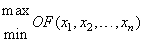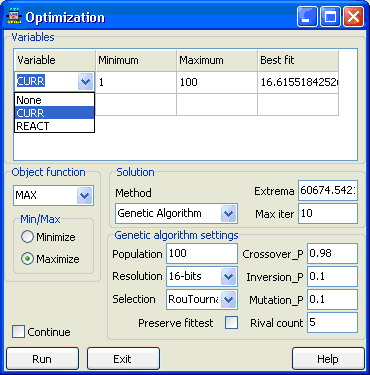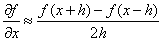﻿ The graphical user interface > Main Window > ATP menu > Optimization

# Optimization

Navigation:  The graphical user interface > Main Window > ATP menu >

# Optimization

The Optimization module runs ATP to evaluate a single cost function and adjust circuit variables based on three different optimization routines (Gradient Method, Genetic algorithm, Simplex Annealing). In order to use the module the cost function Models|WriteMaxMin has to be added to the circuit and variables declared under ATP|Settings/Variables. The variables have to be linked to data in the circuit so that the cost function, OF, is modified with the variables (x1..xn).Fig. 1 - Optimization dialog.

The user first has to select the variables by clicking in the Variables column and select one in the drop down list that appears. Then minimum and maximum constraints must be specified. The best fit column can contain the starting point and it is continously updated as the optimization progesses. Leaving the field blank will cause the average value of the minimum and maximum to be used as starting point.

The user then has to select a cost function (if only one is present it is selected as default) and specify weather to maximize or minimize it. The user also has to select the optimization method and give the maximum number of iterations. It is possible to press ESC to terminate a simulation. In general the Gradient Method is preferred for simple, convex problems (without local minima) or for fine-tuning a solution found by the other two methods. The Genetic Algorithm is good at arriving at an approximate, fair solution, while the Simplex Annealing method potentially can obtain global minimas, allthough slowly. Both the latter two methods require several parameter settings based on experience.

Cost function

The cost function takes in as default a single variable x and writes the maximum or minimum of this value (xout) after the time Tlimit to the lis-file with the syntax:

if T=stoptime

then

write('BEGIN WRITE @UseAS')    --Do not modify this line, replaced by ATPDraw

write(xout,' ',AsFuncOf)       --Add multiple columns or multiple lines

write('END WRITE @UseAS')      --Do not modify this line, replaced by ATPDraw

endif

After the ATP simulation the cost function component reads the results from the lis-file and store it in memory. Multiple run with Pocket Calculator is supported (ATP|Settings/Variables-Number of simulations). The Data parameter AsFuncOf is as default written in a second column and is used as the x-axis when plotting the result under View. If a number is specified in the the AsFuncOf field, the simulation number is simply used instead. Only the first column containing the xout variable is used by the optimization module.

The user can modify the cost function and add more input variables. These have then to be processed in the Model to create a single output variable xout.

Optimization routines

The Gradient Method (GM) is the L-BFGS-B routine  (limited memory algorithm for bound constrained optimization) which is a quasi-Newton method with numerical calculation of the gradient. The gradient is calculated based on the two point formula:where the discretization point h is calculated as  h=max(|x|,1e-6)*dx where dx is a user selectable parameter (delta X).

If n is the number of variables in the optimization problem the cost function thus has to be evaluated 2n+1 times for each solution point. This is done with a single call to ATP utilizing Pocket Calculator.

The user selects two parameters: eps_x (relative convergence criterion) and delta_x (relative derivative increment).

The iteration number is somewhat loosely defined in the Gradient Method. If the solution is poorer than the previous point the algorithm steps backwards along the gradient until an improved solution is found and only then the iteration number is incremented. In general there might be challenges related to different dimensions of variables and some external per unit scaling is recommended. The Gradient Method is also used in the Hybrid Transformer core optimization.

The Genetic Algorithm (GA) is based on the RiverSoft AVG package , but modified to better handle the variable constraints. The development of the solution with GA is to more or less randomly select solutions (individuals) and mate these to obtain new solutions. The selection process can be Random, Roulette (using cumulative distribution), Tournament (competition between a user selectable number of randomly selected rivals), Stochastic Tournament (combination of Roulette and Tournament), and Elitism (select only the user defined best percentage of the population). Tournament with 2-10 rivals is a reasonable starting point. A large number of rivals will approach strong elitism and possible degenerated solutions. Elitism can be selected towards the end of the optimization process.

The Crossover probability (gene based on parents) should be close to, but less than unity (a low number results in cloning). Mutation (random) and Inversion (from parents) should be low, but increased for difficult problems (several local extrema). High numbers will slow down the convergence considerably. The Preserve fittest option will simply copy the fittest individual to the next generation (weak elitism).

The user has to select the size of the population (maximum 1000) and this is a critical parameter which depends on the problem and the number of variables. A population count of 100 should be sufficient for problems with ~2 variables. The Pocket Calculator option of ATP is used for parallel execution (typically ~25) restricted by the limit of intermediate variables in ATP.

The user must also select the resolution with 8, 16 and 32 bits available. This part needs further development to allow integer values and arbitrary resolutions. A resolution of 8 bits (255 levels) is sufficient to reach at a reasonable accurate solution. GM can be used subsequently for fine-tuning.

The GA method does not have a convergence tolerance at the present implementation but will develop for all specified iterations. ESC can be pressed to stop the development, Continue checked to continue it.The Population count and Resolution can not be changed in the optimization process (Continue).

The Simplex Annealing (SA) method is implemented from . It is based on the Nelder-Mead simplex algorithm with an added random behavior gradually reduced (simulated annealing). The algorithm also uses a possible larger set of points (called population) and can support mutation. With all control parameters set to zero the algorithm simply reduces to the classical Nelder-Mead simplex method.

For the Simplex Annealing method the user has to choose the Population (number of points evaluated for membership in the simplex) which is internally restricted to Population=max (Population, n+1). The Mutation probability parameter controls if the new points in the simplex is found at random or with the classical methods reflection, expansion or contradiction. The Max Climbs parameter controls how many steps in a negative direction that is accepted by the method. This should be a moderate value 0-3. The parameter beta (<1) controls annealing schedule (temperature reduction), and the parameter ratio (controls the annealing schedule when a local minimum is found.  For a rough surface with many local minima the beta and ration parameters needs to be increased. Ftol is the convergence criterion (the downside of this method). The iteration stops if.

The method relies only on function evaluations and POCKET CALCULATOR of ATP is thus not used. Since a single case is run through ATP for each cost function evaluation, the method thus has potential to be extended to include other variables than those defined within the global variables.

The optimization module was established in co-operation with Schneider Electric, France.

1.C. Zhu, R.H. Byrd and J. Nocedal : L-BFGS-B: Algorithm 778: L-BFGS-B, FORTRAN routines for  large  scale  bound  constrained  optimization, ACM Transactions on Mathematical Software,  Vol 23,  Num. 4, 1997 Page(s): 550 - 560.

3.W. H. Press, S. A. Teukolsky, W. T. Vetterling, B. P, Flannery: Numerical recipes, 2nd Ed. 1992, Cambridge University Press.